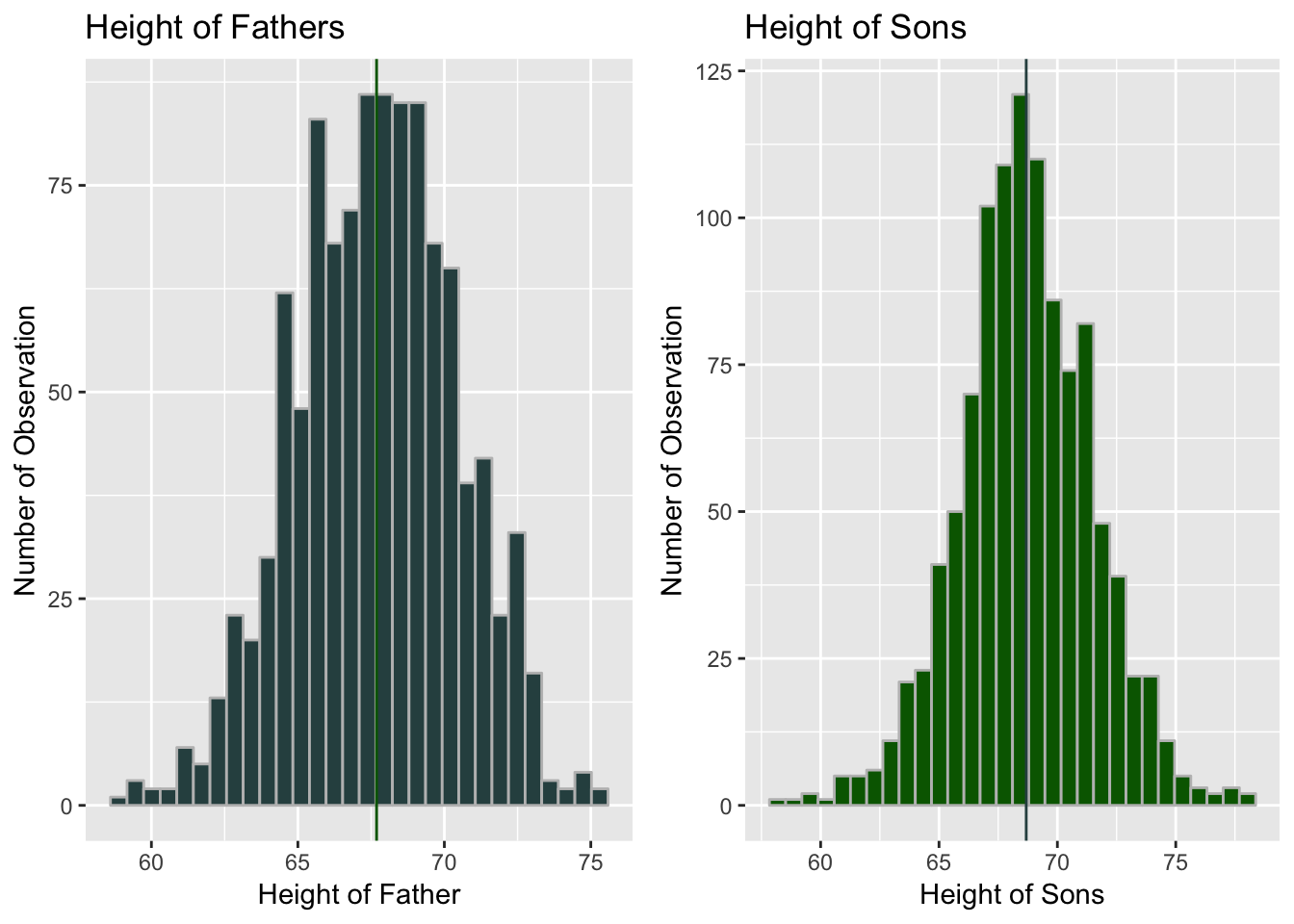# Father and Son Analysis

Father and son dataset is obtained from the study of Sir Francis Galton on the hieght of fathers and their sons. The dataset contains height of 1078 fathers and their corresponding sons in inches. The analysis will establish a linear relation between height of father and sons. Furthermore, through a linear regression model, the height of son is predicted by height of fathers.

### Exploratory Data Analysis

We obtain the data from the UsingR package of R library.

``````# Exploring the dataset
``````##    fheight  sheight
## 1 65.04851 59.77827
## 2 63.25094 63.21404
## 3 64.95532 63.34242
## 4 65.75250 62.79238
## 5 61.13723 64.28113
## 6 63.02254 64.24221``````
``dim(father.son)``
``##  1078    2``
``str(father.son)``
``````## 'data.frame':    1078 obs. of  2 variables:
##  \$ fheight: num  65 63.3 65 65.8 61.1 ...
##  \$ sheight: num  59.8 63.2 63.3 62.8 64.3 ...``````
``````# Rounding up the values to the 2 digit
father.son <- round(father.son, digit = 2)``````
``````library(gridExtra)

# Histogram of height of father

fplot <- ggplot(father.son, aes(x = fheight))+ geom_histogram(col = "grey", fill = "darkslategray") + geom_vline(xintercept = mean(father.son\$fheight), col = "dark green") + xlab("Height of Father") + ylab("Number of Observation") + ggtitle("Height of Fathers")

# Histogram of height of Son
splot <- ggplot(father.son, aes(x = sheight)) + geom_histogram(fill = "dark green", col = "grey") + geom_vline(xintercept = mean(father.son\$sheight), col = "darkslategray") + xlab("Height of Sons") + ylab("Number of Observation") + ggtitle("Height of Sons")

# Scatterplot of the dataset
scatplot <- Fa.son <- ggplot(father.son,  aes(x = fheight, y = sheight)) + geom_point(col = "darkslategray") + xlab("Height of Father") + ylab("Height of Sons") + ggtitle("Height of Fathers and Sons")

grid.arrange(fplot, splot, ncol = 2, nrow = 1)``````
``````## `stat_bin()` using `bins = 30`. Pick better value with `binwidth`.
## `stat_bin()` using `bins = 30`. Pick better value with `binwidth`.````````scatplot``